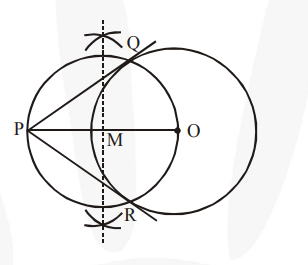# Draw a circle of radius 6 cm. From a point 10 cm away from its centre,

Question:

Draw a circle of radius 6 cm. From a point 10 cm away from its centre, construct the pair of tangents to the circle and measure their lengths.

Solution:

Steps of construction :

1. Taking any point O of the given plane as centre, draw a circle of 6 cm radius. Locate a point P, 10 cm away from O. Join OP.

2. Bisect OP. Let M be the mid-point of PO.

3. Taking M as centre and MO as radius, draw a circle.

4. Let this circle intersect the previous circle at point Q and R.

5. Join PQ and PR. PQ and PR are the required tangents.The lengths of tangents PQ and PR are 8 cm each.